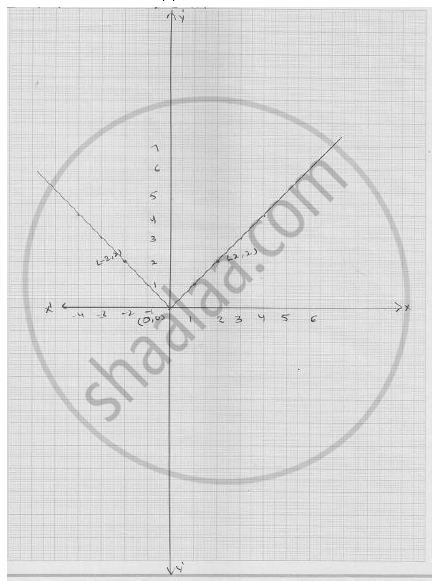# Draw the Graph of Y = | X |. - Mathematics

Draw the graph of y = | x |.

#### Solution

We have

y = | x |      ........... (1)

Putting  x = 0 , we get  y = 0

Putting  x = 2 , we get y = 2

Putting  x = 2 , we get  y  =  - 12

Thus, we have the following table for the two points on graph of   | x |

 x 0 2 -2 y 0 2 2

Graph of line equation y =  | x |Concept: Graph of a Linear Equation in Two Variables
Is there an error in this question or solution?

#### APPEARS IN

RD Sharma Mathematics for Class 9
Chapter 7 Linear Equations in Two Variables
Exercise 7.3 | Q 15

Share